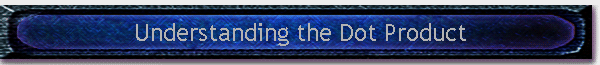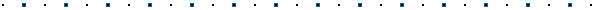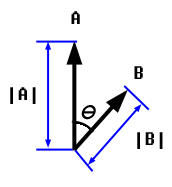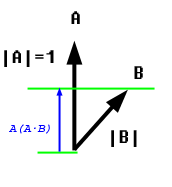#Written by Robert Dunlop Microsoft DirectX MVP### Introduction

The dot product is a value expressing the angular relationship between two vectors.  In this article we will learn how this value is calculated, its mathematical significance, and several ways in which this function is useful in 3D applications.### Calculating the Dot Product

A dot product is a scalar value that is the result of an operation of two vectors with the same number of components.  Given two vectors A and B each with n components, the dot product is calculated as:

A · B = A1B1 + ... + AnBn

The dot product is thus the sum of the products of each component of the two vectors.  For example if A and B were 3D vectors:

A · B = A.x * B.x + A.y * B.y + A.z * B.z

A generic C++ function to implement a dot product on two floating point vectors of any dimensions might look something like this:

```float dot_product(float *a,float *b,int size) {     float dp = 0.0f;     for (int i=0;i<size;i++)         dp += a[i] * b[i];     return dp; }```

This sample code is provided solely for the purpose of showing a generic function to clarify how the dot product is calculated;  DirectX provides several implementations of this function for you, as you will see further on, though if you did need to write your own function (for example if using C++ without the D3DX libraries) you would likely just write separate functions to handle the vector types commonly used (2D,3D,4D) as inline code.### So what does it mean?

Earlier we said that the dot product represents an angular relationship between two vectors, and left it at that.  Now we'll take a closer look at what this value represents.Let's say we have two vectors, A and B, as shown to the left.  The values |A| and |B| represent the lengths of vectors A and B, respectively, and Θ is the angle between the two vectors.  The dot product of vectors A and B will have the following relationship to these values:

A · B = |A| * |B| * cos(Θ)

That is to say, the dot product of two vectors will be equal to the cosine of the angle between the vectors, times the lengths of each of the vectors.

Angular Domain of Dot Product:

Given the characteristics of the cosine function, we can deduce three possible conditions:

1. If A and B are perpendicular (at 90 degrees to each other), the result of the dot product will be zero, because cos(Θ) will be zero.
2. If the angle between A and B are less than 90 degrees, the dot product will be positive (greater than zero), as cos(Θ) will be positive, and the vector lengths are always positive values.
3. If the angle between A and B are greater than 90 degrees, the dot product will be negative (less than zero), as cos(Θ) will be negative, and the vector lengths are always positive values.

Angle from Dot Product of Unit Vectors

The above characteristics are true for any vectors with non-zero length.  In addition, there is a special case when both vectors are unit vectors, that is, vectors with a length of one (1.0).  In this case, the lengths of the vectors does not contribute to the equation, simplifying to:

A · B = |A| * |B| * cos(Θ)
A · B = 1 * 1 * cos(Θ)
A · B = cos(Θ)

In this case, the dot product is equal to the cosine of the angle between the vectors.  Thus the angle between unit vectors can be calculated as:

Θ = acos(A · B)

Angle from Dot Product of Non-Unit Vectors

Angles between non-unit vectors (vectors with lengths not equal to 1.0) can be calculated either by first normalizing the vectors, or by dividing the dot product of the non-unit vectors by the length of each vector.

Dot Product of Vector with Itself

Taking the dot product of a vector against itself (i.e. A · A) results in a value equal to the square of the vector's length.  This is a familiar portion of the distance equation, d=sqrt(x*x+y*y+z*z).

Projection of Vector onto another VectorIf one were to take the dot product of a unit vector A and a second vector B of any non-zero length, the result is the length of vector B projected in the direction of vector A (see illustration to left).  This is used in a number of ways, such as collision response and conversion of vectors from one coordinate system to another (this forms the basis of matrix transformations).### DirectX Implementations

DirectX Graphics provides several implementations of the dot product function:

 Programming in... Functions Provided C++ FLOAT D3DXVec2Dot(const D3DXVECTOR2 *,const D3DXVECTOR2 *) FLOAT D3DXVec3Dot(const D3DXVECTOR3 *,const D3DXVECTOR3 *) FLOAT D3DXVec4Dot(const D3DXVECTOR4 *,const D3DXVECTOR4 *) Managed DX Microsoft.DirectX.Vector2.Dot(Vector2,Vector2) Microsoft.DirectX.Vector3.Dot(Vector3,Vector3) Microsoft.DirectX.Vector4.Dot(Vector4,Vector4) HLSL Shader Language dot(vector,vector) mul(vector,vector) Vertex Shader (Assembly) dp3 dest,src0,src1 dp4 dest,src0,src1 Pixel Shader (Assembly) dp2add dest,src0,src1,src2.{x|y|z|w} dp3 dest,src0,src1 dp4 dest,src0,src1This site, created by DirectX MVP Robert Dunlop and aided by the work of other volunteers, provides a free on-line resource for DirectX programmers.

Special thanks to WWW.MVPS.ORG, for providing a permanent home for this site.

#####Visitors Since 1/1/2000:Last updated: 07/26/05.